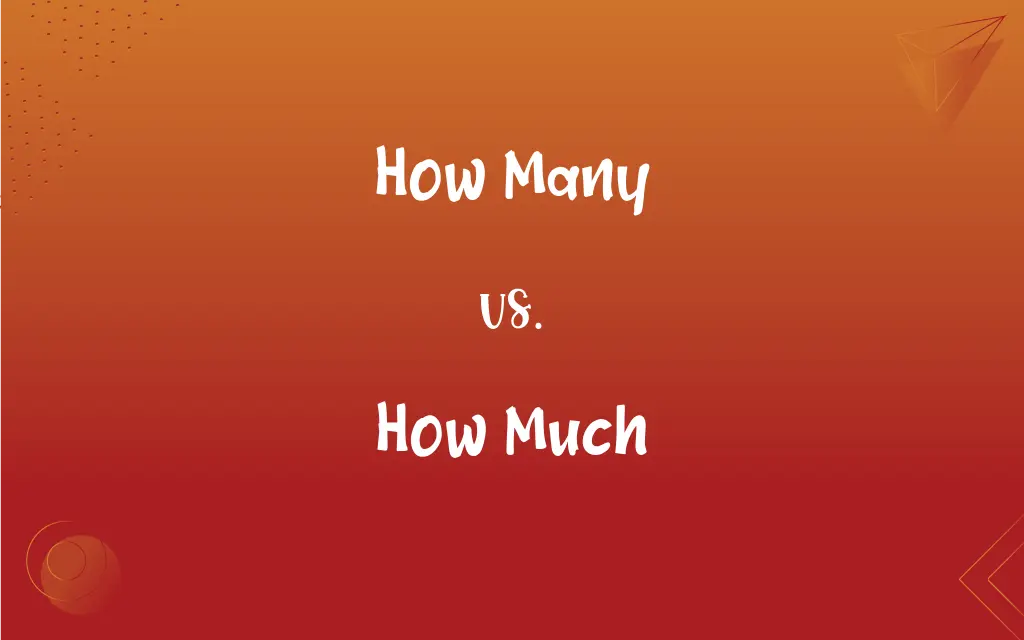# How Many vs. How Much: What's the Difference?Edited by Huma Saeed || By Sawaira Riaz || Published on November 19, 2023
"How Many" queries the count of discrete items, while "How Much" asks about the quantity or extent of uncountable or abstract entities.## Key Differences

"How Many" and "How Much" are both questions of quantity, but they cater to different kinds of nouns and concepts.
"How Many" is used when referring to countable nouns. For instance, when we ask about the number of books on a shelf, we say, "How many books are there?" It deals with individual, separate items that can be enumerated. In contrast, "How Much" relates to uncountable nouns or those things that don't have a distinct separation. When asking about the volume of water in a bottle, one would ask, "How much water is there?" It delves into matters of degree, extent, or volume, often pertaining to things that come in a mass, volume, or abstract quantity
. Another way to look at it is in terms of specificity. "How Many" seeks a specific number as an answer, while "How Much" typically expects a measure, value, or general amount.

## Comparison Chart

### Nature

Countable
Uncountable

Specific number
Measure, value, or general amount

### Refers To

Discrete items
Mass, volume, degree, or abstract quantity

### Common Usage

Questions about volume, extent, or degree

### Example Question

How many apples do you have?
How much juice do you have?

## How Many and How Much Definitions

#### How Many

An interrogation about the sum of particular things.
How many miles did you run?

#### How Much

An interrogation about the general amount or degree.

#### How Many

An inquiry into the specific count of entities.
How many players are on the team?

#### How Much

A question seeking the measurement or value of an entity.
How much does this cost?

#### How Many

A probe into the quantity of specified items.
How many cookies did you eat?

#### How Much

An inquiry into the extent or volume of something.
How much space does this take?

#### How Many

A question about the total of distinct units.
How many days are in a week?

#### How Much

A probe into the level or magnitude of a concept.
How much time do we have left?

#### How Many

A query regarding the number of countable items.
How many pencils are in the box?

#### How Much

A query about the quantity or degree of uncountable entities.
How much water do you drink daily?

## FAQs

#### Can "How Much" refer to money?

Yes, like "How much does it cost?"

#### Why can't I say "How much apples"?

"Apples" are countable, so "How many apples?" is correct.

#### When is "How Much" appropriate?

Use it for uncountable nouns or abstract quantities.

#### Can "How Much" be about emotions?

Yes, like "How much do you love me?"

#### Is "How Many" for plural nouns?

Yes, it's for plural countable nouns.

#### Which is for liquids, "How Many" or "How Much"?

"How Much" is for liquids, like water or juice.

#### Is "How Many" always about specific numbers?

Mostly, it seeks a defined count.

#### Why is it "How many pieces" but "How much cake"?

"Pieces" are countable, "cake" in bulk isn't.

#### Can "How Many" refer to frequency?

Yes, like "How many times have you been here?"

#### Can "How Much" be used for time?

Yes, as in "How much time did it take?"

#### Can "How Much" refer to size?

Yes, like "How much bigger is it?"

#### Can I use "How Much" for distance?

Yes, like "How much farther is it?"

#### Can "How Many" refer to parts of a whole?

Yes, like "How many slices of the pie?"

#### Is it "How many sugar" or "How much sugar"?

It's "How much sugar?" as sugar is uncountable.

#### Is "How Much" for abstract concepts?

Often, as in "How much knowledge do you have?"

#### Is "How many air" correct?

No, use "How much air?" as air is uncountable.

#### Is it "How many milk" or "How much milk"?

It's "How much milk?" as milk is uncountable.

#### Can "How Much" be about effort?

Yes, like "How much effort did you put in?"

#### Which should I use for collective nouns?

Typically "How much," like "How much luggage?"